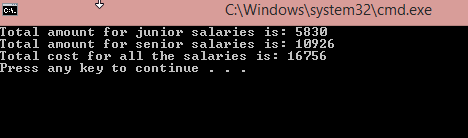The Strategy design pattern is a behavioral design pattern that allows us to define different functionalities, put each functionality in a separate class and make their objects interchangeable.

In other words, we have a main Context object that holds a reference towards a Strategy object and delegates it by executing its functionality. If we want to change the way the Context performs its work, we can just replace the current Strategy object with another one.

So without further ado, let’s dive deeper into the Strategy design pattern implementation.

The source code is available at the Strategy Design Pattern – Source Code.

For the main page of this series check out C# Design Patterns.

## Strategy Design Pattern Structure

As we stated above, the Strategy design pattern consists of the Context object which maintains the reference towards the strategy object. But it is not the only part of the puzzle. For the complete implementation, we need the Strategy object (interface) to define a way for the Context object to execute the strategy and the Concrete Strategies objects which implement the Strategy interface.

The Strategy design pattern is quite common in the C# language due to its various uses to provide changing behavior of a class without modifying it. This complies with the rules of the Open Closed Principle, which we talked about in one of our previous articles.

## Implementation of the Strategy Design Pattern

To implement the Strategy pattern, we are going to reuse an example from our Open Closed Principle article.

So, the main task is to calculate the total cost for the developer’s salaries, but for the different developer levels, the salary is calculated differently. Now, we are going to modify that example by introducing the Strategy design pattern.

So let’s start with the `DeveloperLevel` enumeration and a simple `DeveloperReport` object:

```public enum DeveloperLevel
{
Senior,
Junior
}
```
```public class DeveloperReport
{
public int Id { get; set; }
public string Name { get; set; }
public DeveloperLevel Level { get; set; }
public int WorkingHours { get; set; }
public double HourlyRate { get; set; }

public double CalculateSalary() => WorkingHours * HourlyRate;
}
```

To continue on, let’s create the Strategy interface named `ISalaryCalculator`:

```public interface ISalaryCalculator
{
double CalculateTotalSalary(IEnumerable<DeveloperReport> reports);
}
```

Now what we need is the concrete strategy objects which will accept all the reports and calculate the total salary for the required developer levels:

```public class JuniorDevSalaryCalculator : ISalaryCalculator
{
public double CalculateTotalSalary(IEnumerable<DeveloperReport> reports) =>
reports
.Where(r => r.Level == DeveloperLevel.Junior)
.Select(r => r.CalculateSalary())
.Sum();
}
```
```public class SeniorDevSalaryCalculator : ISalaryCalculator
{
public double CalculateTotalSalary(IEnumerable<DeveloperReport> reports) =>
reports
.Where(r => r.Level == DeveloperLevel.Senior)
.Select(r => r.CalculateSalary() * 1.2)
.Sum();
}
```

As we can see, for the senior developers, we are adding a 20% bonus to the salary. Additionally, we have separated our calculation logic for the different developer levels and made easier to add calculation logic for the medior developers for example. All we would have to do is to add an additional strategy object which implements the `ISalaryCalculator` interface.

Once we have the strategy objects, we need the context object in our implementation:

```public class SalaryCalculator
{
private ISalaryCalculator _calculator;

public SalaryCalculator(ISalaryCalculator calculator)
{
_calculator = calculator;
}

public void SetCalculator(ISalaryCalculator calculator) => _calculator = calculator;

public double Calculate(IEnumerable<DeveloperReport> reports) => _calculator.CalculateTotalSalary(reports);
}
```

In this context object, we provide initialization of the strategy object with the constructor in a compile-time or with the `SetCalculator` method in the application’s runtime. Furthermore, the `Calculate` method just executes the strategy object functionality.

So, to connect all the dots together, let’s modify the `Program.cs` class:

```class Program
{
static void Main(string[] args)
{
var reports = new List<DeveloperReport>
{
new DeveloperReport {Id = 1, Name = "Dev1", Level = DeveloperLevel.Senior, HourlyRate = 30.5, WorkingHours = 160 },
new DeveloperReport { Id = 2, Name = "Dev2", Level = DeveloperLevel.Junior, HourlyRate = 20, WorkingHours = 120 },
new DeveloperReport { Id = 3, Name = "Dev3", Level = DeveloperLevel.Senior, HourlyRate = 32.5, WorkingHours = 130 },
new DeveloperReport { Id = 4, Name = "Dev4", Level = DeveloperLevel.Junior, HourlyRate = 24.5, WorkingHours = 140 }
};

var calculatorContext = new SalaryCalculator(new JuniorDevSalaryCalculator());
var juniorTotal = calculatorContext.Calculate(reports);
Console.WriteLine(\$"Total amount for junior salaries is: {juniorTotal}");

calculatorContext.SetCalculator(new SeniorDevSalaryCalculator());
var seniorTotal = calculatorContext.Calculate(reports);
Console.WriteLine(\$"Total amount for senior salaries is: {seniorTotal}");

Console.WriteLine(\$"Total cost for all the salaries is: {juniorTotal+seniorTotal}");
}
}
```

This should be our result:## When Should We Use the Strategy Design Pattern

We should use this pattern whenever we have different variations for some functionality in an object and we want to switch from one variation to another in a runtime. Furthermore, if we have similar classes in our project that only differ on how they execute some behavior, the Strategy pattern should be the right choice for us.

We should consider introducing this pattern in situations where a single class has multiple conditions over different variations of the same functionality. That’s because the Strategy pattern lets us extract those variations into separate classes (concrete strategies). Then we can invoke them into the context class.

## Conclusion

As you can see, implementing the Strategy design pattern isn’t hard or complex at all.

It makes our code more readable and easier to maintain. Yes, it requires an implementation of additional classes in the project, but that’s the price worth paying for.

So, we have learned:

• What is the Strategy design pattern
• How to implement it in C#
• And, when to use it